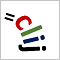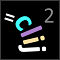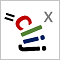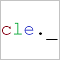# CLIJ2GPU accelerated image processing for everyone

CLIJ2 homeCalculates the sum of pairs of pixels x and y from images X and Y weighted with factors a and b.

`f(x, y, a, b) = x * a + y * b`

### Parameters

summand1 : Image The first input image to added. summand2 : Image The second image to be added. destination : Image The output image where results are written into. factor1 : float The constant number which will be multiplied with each pixel of summand1 before adding it. factor2 : float The constant number which will be multiplied with each pixel of summand2 before adding it.

Category: Math

Availability: Available in Fiji by activating the update sites clij and clij2. This function is part of clij2_-2.5.0.1.jar.

### Usage in ImageJ macro

``````Ext.CLIJ2_addImagesWeighted(Image summand1, Image summand2, Image destination, Number factor1, Number factor2);
``````

### Usage in object oriented programming languages

Java
```// init CLIJ and GPU
import net.haesleinhuepf.clij2.CLIJ2;
import net.haesleinhuepf.clij.clearcl.ClearCLBuffer;
CLIJ2 clij2 = CLIJ2.getInstance();

// get input parameters
ClearCLBuffer summand1 = clij2.push(summand1ImagePlus);
ClearCLBuffer summand2 = clij2.push(summand2ImagePlus);
destination = clij2.create(summand1);
float factor1 = 1.0;
float factor2 = 2.0;
```
```// Execute operation on GPU
```
```// show result
destinationImagePlus = clij2.pull(destination);
destinationImagePlus.show();

// cleanup memory on GPU
clij2.release(summand1);
clij2.release(summand2);
clij2.release(destination);
```
Matlab
```% init CLIJ and GPU
clij2 = init_clatlab();

% get input parameters
summand1 = clij2.pushMat(summand1_matrix);
summand2 = clij2.pushMat(summand2_matrix);
destination = clij2.create(summand1);
factor1 = 1.0;
factor2 = 2.0;
```
```% Execute operation on GPU
```
```% show result
destination = clij2.pullMat(destination)

% cleanup memory on GPU
clij2.release(summand1);
clij2.release(summand2);
clij2.release(destination);
```
Icy JavaScript
```// init CLIJ and GPU
importClass(net.haesleinhuepf.clicy.CLICY);
importClass(Packages.icy.main.Icy);

clij2 = CLICY.getInstance();

// get input parameters
summand1_sequence = getSequence();
summand1 = clij2.pushSequence(summand1_sequence);
summand2_sequence = getSequence();
summand2 = clij2.pushSequence(summand2_sequence);
destination = clij2.create(summand1);
factor1 = 1.0;
factor2 = 2.0;
```
```// Execute operation on GPU
```
```// show result
destination_sequence = clij2.pullSequence(destination)
// cleanup memory on GPU
clij2.release(summand1);
clij2.release(summand2);
clij2.release(destination);
```
clEsperanto Python (experimental)
```import pyclesperanto_prototype as cle